The next step in the univariate FSSA algorithm for the call center fts object is to find the principal components of the data. These principal components are the main building blocks of what make up the call center fts object. They are orthonormal to one another and will be used for reconstruction to approximate the original fts object. One could think of this step as denoising the data and trying to find the most informative pieces of the data that expose trend, seasonality, and other components that will be interesting. We achieve this by performing a singular value decomposition (SVD) of the trajectory operator that results from the embedding step of FSSA which is also performed as a part of the ‘fssa’ function.

For our univariate call center example, we choose a lag of 28 to try to expose the daily pattern of call center data within a week (4 weeks in a month with 7 days in a week).

L=28
U=fssa(Y,L)

We obtain the functional singular spectrum analysis (fssa) object, U, which contains the principal components (eigenfunctions) and all the additional information that comes with those components such as associated eigenvalues. With our components, we want to reconstruct into additive fts objects whose sum approximates the original fts object but without the noise components involved. We also want to do the reconstructions such that we separate out the trend term from the periodic term and so forth. This grouping of fssa objects can be done heuristically by looking at plots of the decomposition. For our call center example, we start with the scree plot of singular values.

These singular values capture how much energy or information is stored within each prinicipal component. We see that the first component contains most of the variation in the data and subsequent components contain less variation in the data. Notice that the second and third singular values are similar in magnitude, this is a clue that we may want to group the second and third components together for reconstruction.

plot(U,d=13)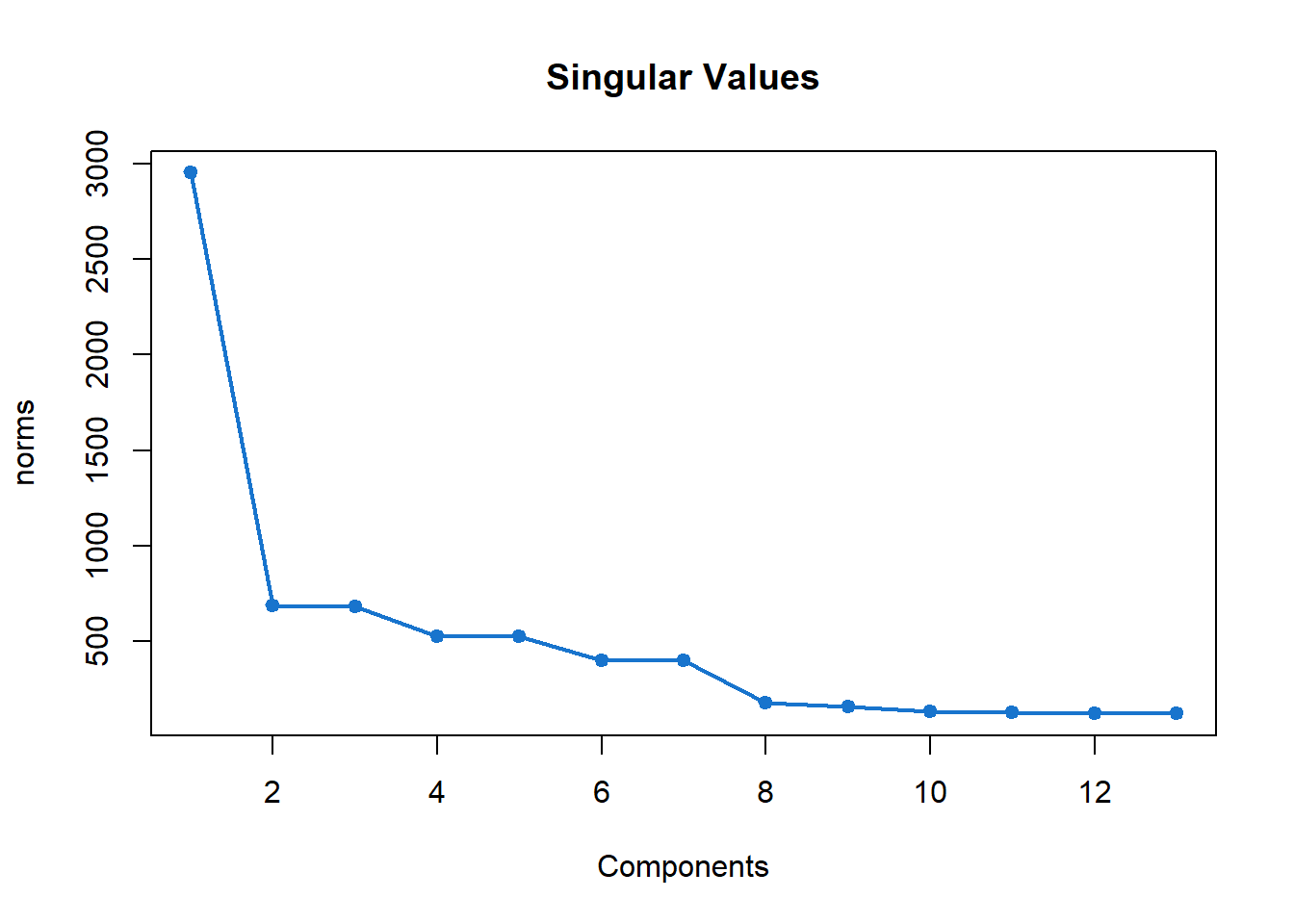This plot is a heat map of the principal components and shows periodic patterns that are present in each of the components. Again, we see similar periodic behavior between the second and third components strengthening our argument to group these two together for reconstruction. We see four and five also have a similar pattern compared to each other and the same goes for six and seven.

plot(U,d=9,type="lheats")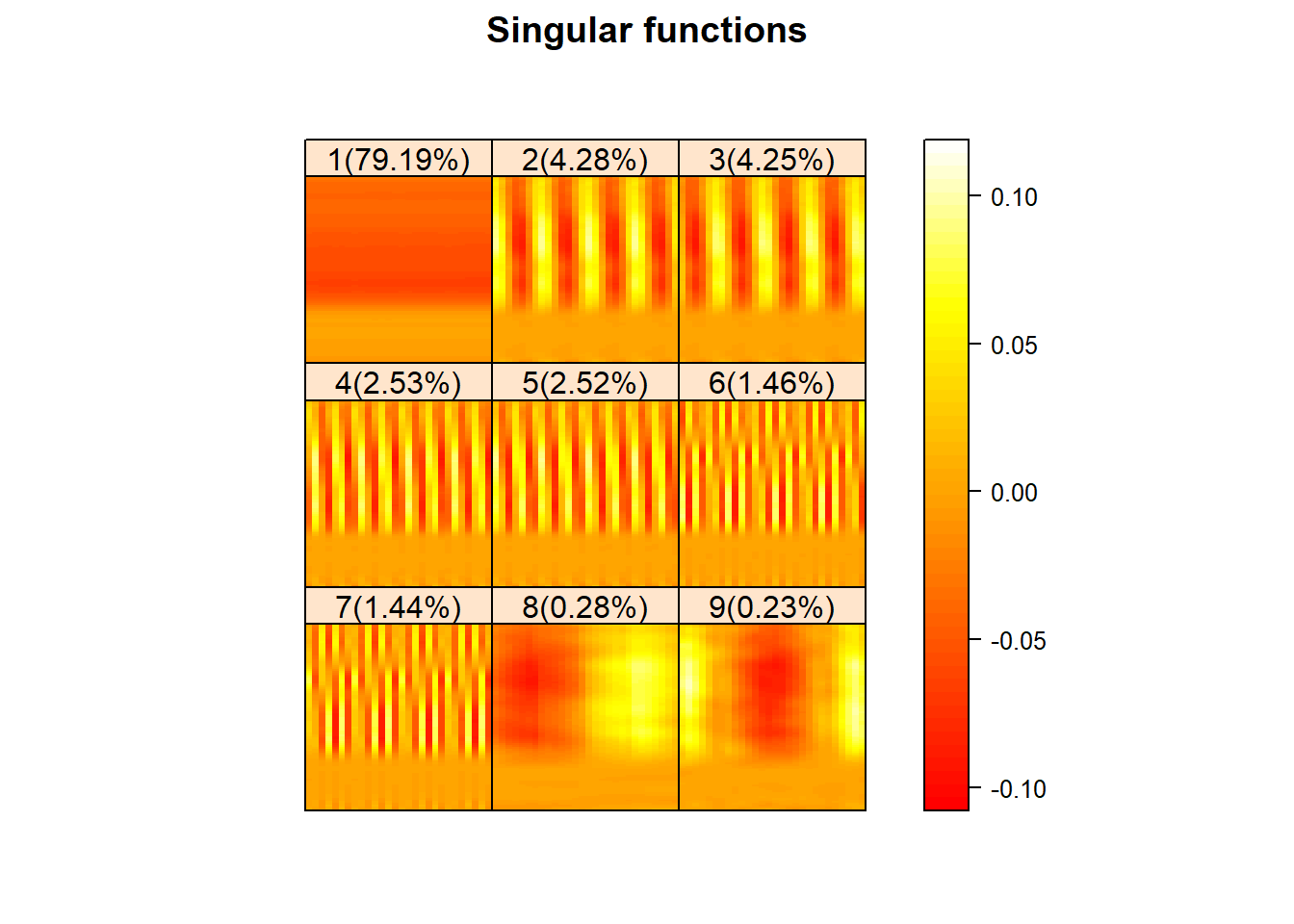This plot is of the principal components which gives us information into what types of behaviors they best capture. We see that the first component seems to capture some overarching trend while the second, third, fourth, fifth, sixth, and seventh seem to all capture some sort of daily periodic behavior as expected. Each of these components have seven distinct curves of information which tells us that they are capturing information about calls to the center on each of the seven days of the week.

plot(U,d=9,type="lcurves")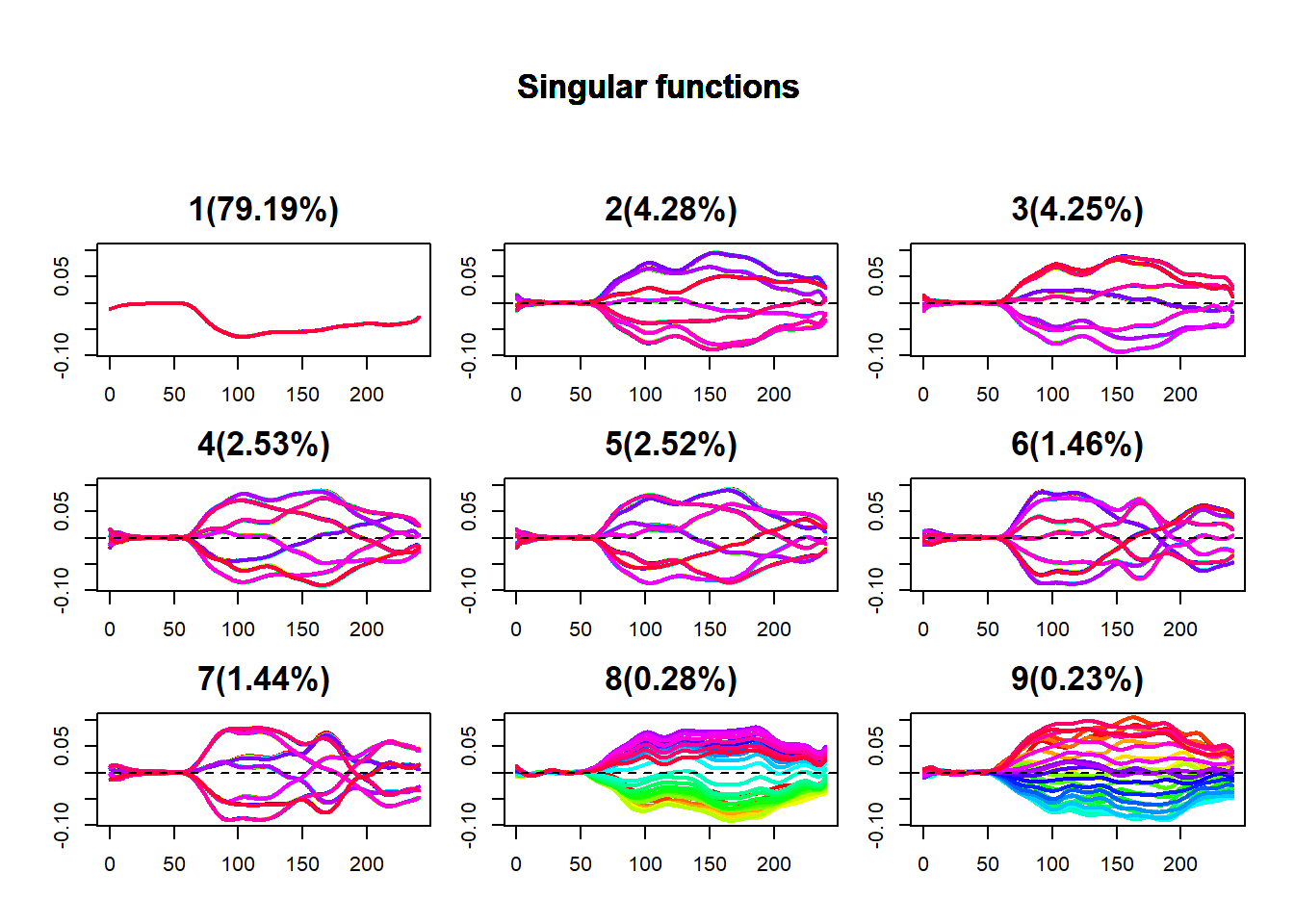This is a plot of the right singular vectors which can capture periodic behavior within components. This plot tells us that there is periodic component of about 10 time units present within each daily function which is roughly our sampling frequency.

plot(U,d=9,type="vectors")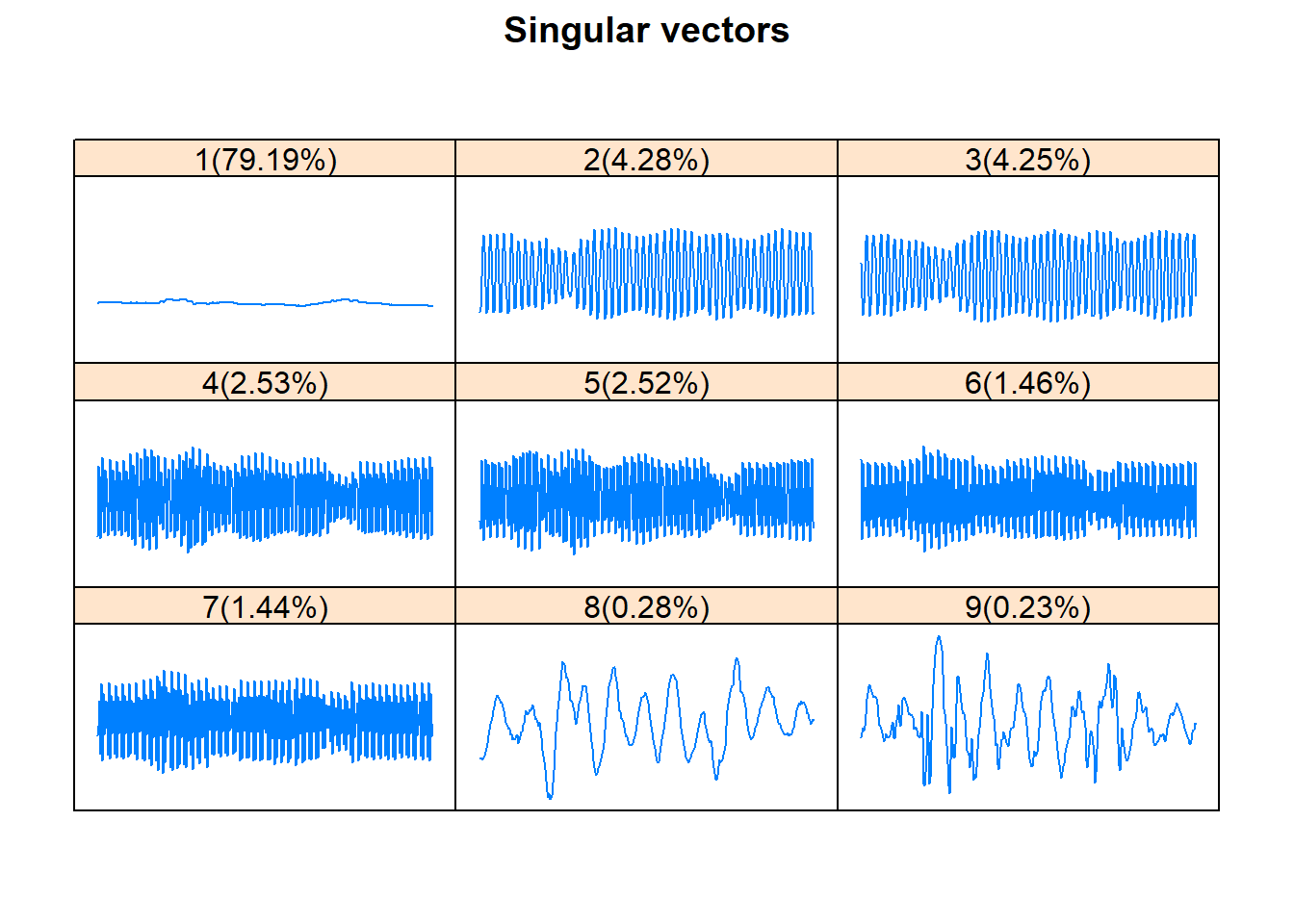This periodogram plot is a way to detect periodic behavior in the fts object between observations in the fts object. For the callcenter data, we have roughly a seven day periodic behavior between days.

plot(U,d=9,type="periodogram")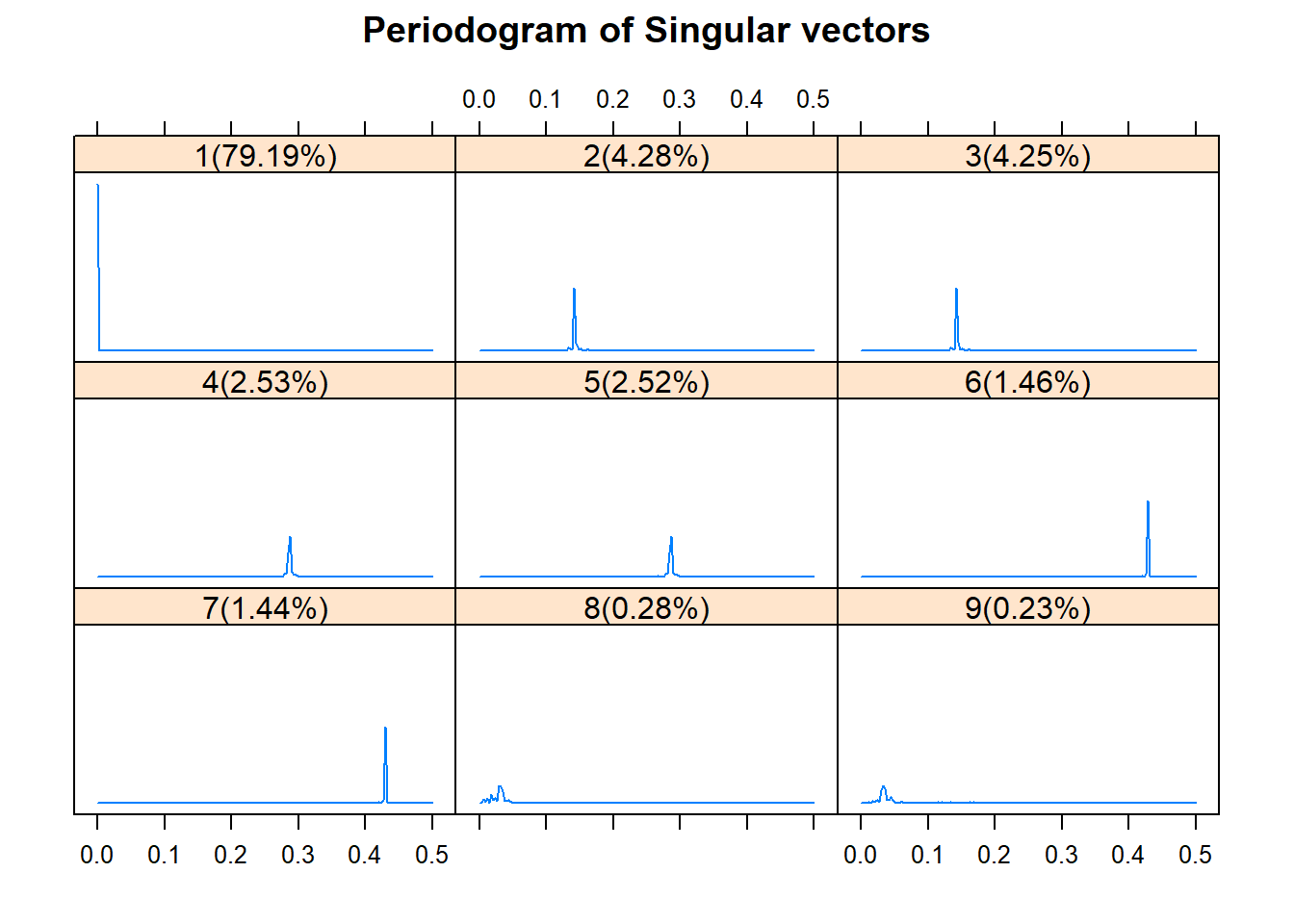The paired plot exposes how principal components behave with one another especially in a periodic sense. If principal components form a nice geometry with one another when plotted together, then a nice grouping is exposed and in addition, the geometry tells us what type of periodicity those principal components capture. For this call center example, we see that the second and third component have a nice geometry, four and five also have a nice geometry, and so do six and seven. Each one of these geometric shapes have seven points which indicates they are capturing daily behavior of calls received by the center during each of the distinct seven days of the week.

plot(U,d=9,type="paired")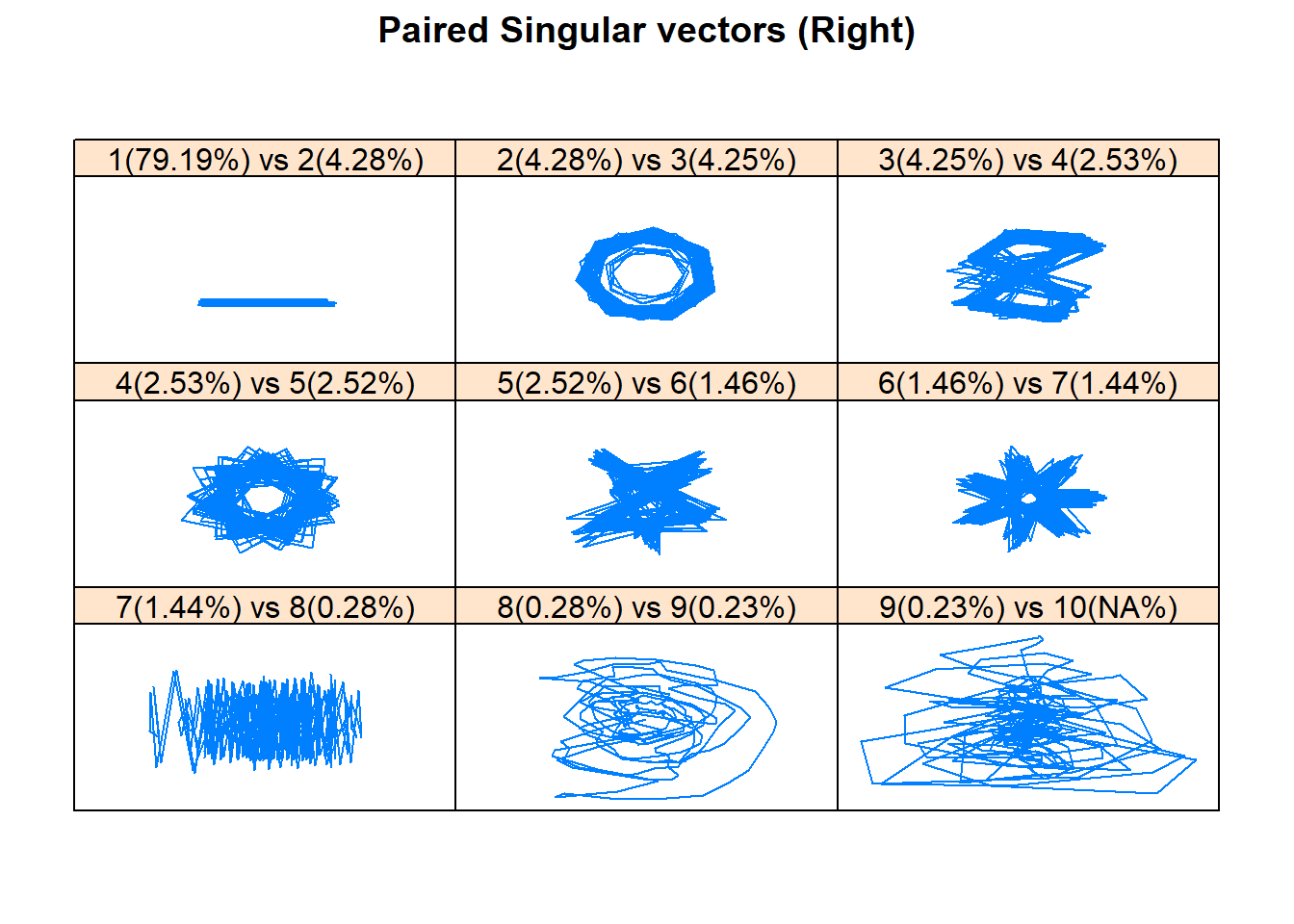In addition to these plots of principal components, can analyze the weighted correlation (w-correlation) matrix plot which showcases the correlation of fts objects that are formed from reconstructions using each principal component individually in the grouping. The plot can be used to make decisions on an appropriate grouping and it also makes it easy to distinguish useful components from components that only capture noise. Darker groupings of squares indicate a higher level of correlation between the fts objects built from those principal components and thus, those components should be grouped together for reconstruction. We form the w-correlation matrix and then plot it with the following.

W = fwcor(U,group=list(1,2,3,4,5,6,7,8,9,10,11,12,13,14,15,16,17,18,19,20)) #Find the weighted w-correlation matrix
wplot(W,cuts = 14)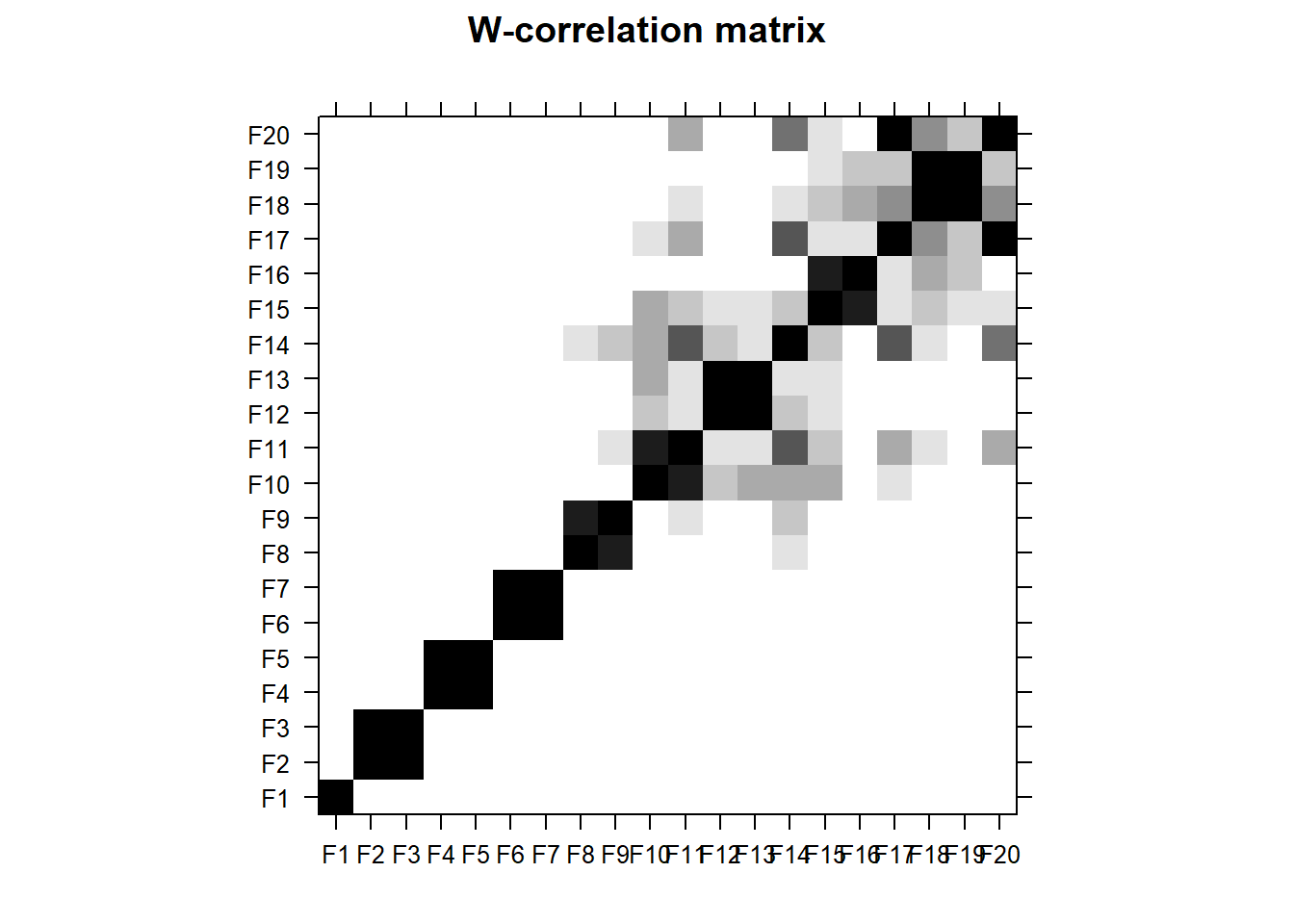For the call center data example, we again see that one should be grouped by itself, two with three, four with five, and six with seven. We also see that the eight component and subsequent components that come after it capture noise of the fts object. The ‘cuts’ parameter was used to make visualization of the matrix easier.

The ‘plot’ function can also be used to create a plot of the w-correlation matrix. Setting the ‘d’ parameter to be 20, we obtain the following w-correlation matrix without the ‘cuts’ parameter which made visualization easier.

plot(U,d=20,type="wcor")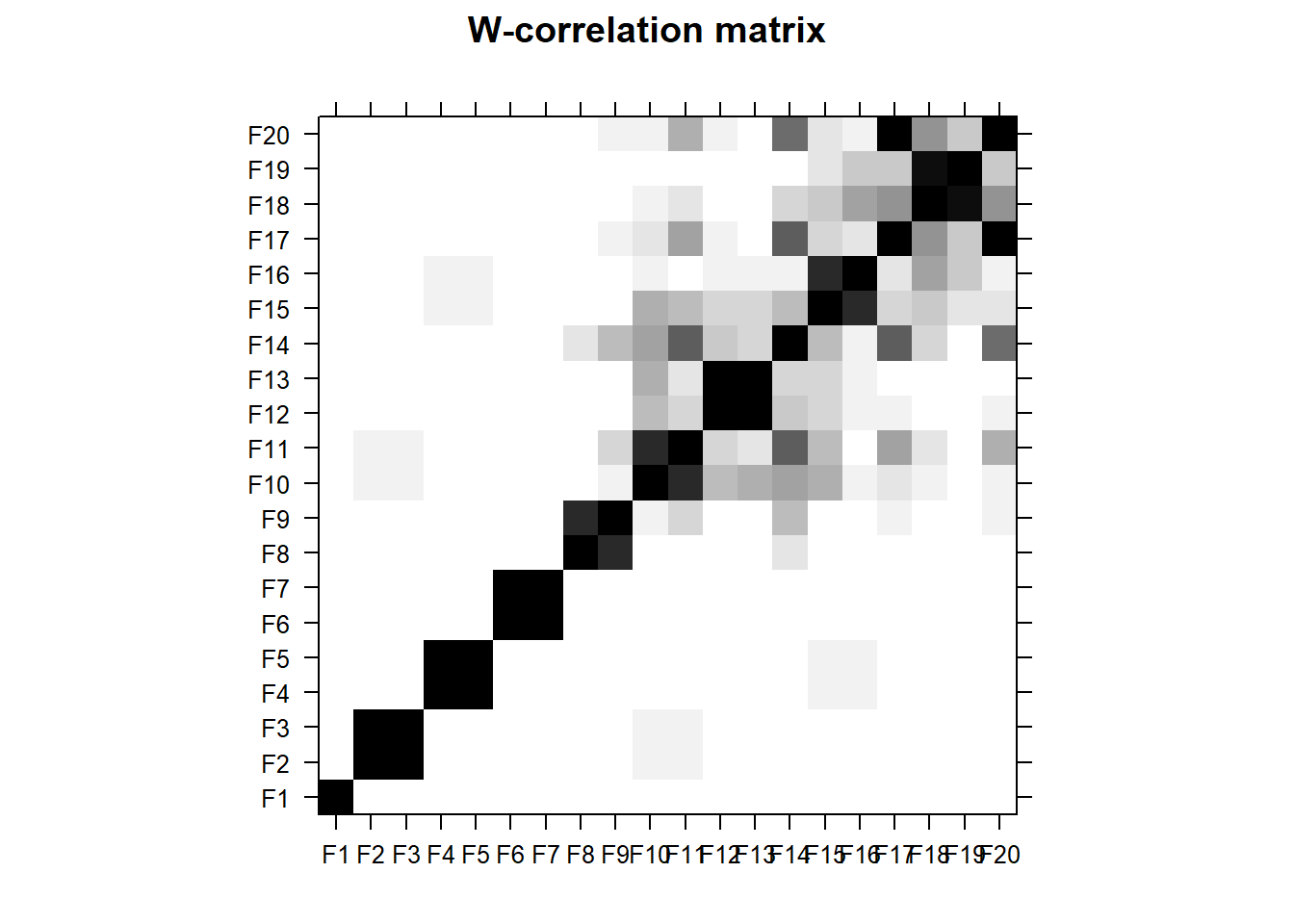Now that we have our suitable grouping and we have identified the general trend and periodic components of the call center fts object. We can reconstruct into additive fts objects.